# InitialConditions

Setting of initial conditions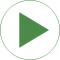# Information

This information is part of the Modelica Standard Library maintained by the Modelica Association.

There are several ways to set initial conditions. In the first system the position of the mass m3 was defined by using the modifier s(start=4.5), the position of m4 by s(start=12.5). These positions were chosen such that the system is at rest. To calculate these values start at the left (fixed2) with a value of 1 m. The spring s2 has an unstretched length of 2 m and m3 an length of 3 m, which leads to

```  1   m (fixed2)
+ 2   m (spring s2)
+ 3/2 m (half of the length of mass m3)
-------
4,5 m = s(start = 4.5) for m3
+ 3/2 m (half of the length of mass m3)
+ 4   m (springDamper sd2)
+ 5/2 m (half of length of mass m4)
-------
12,5 m = s(start = 12.5) for m4
```

This selection of initial conditions can prioritize the selection of those variables (m3.s and m4.s) as state variables.

In the second example, the lengths of the springs are given start values but they cannot be used as state for pure springs (only for the spring/damper combination). In this case the system is not at rest.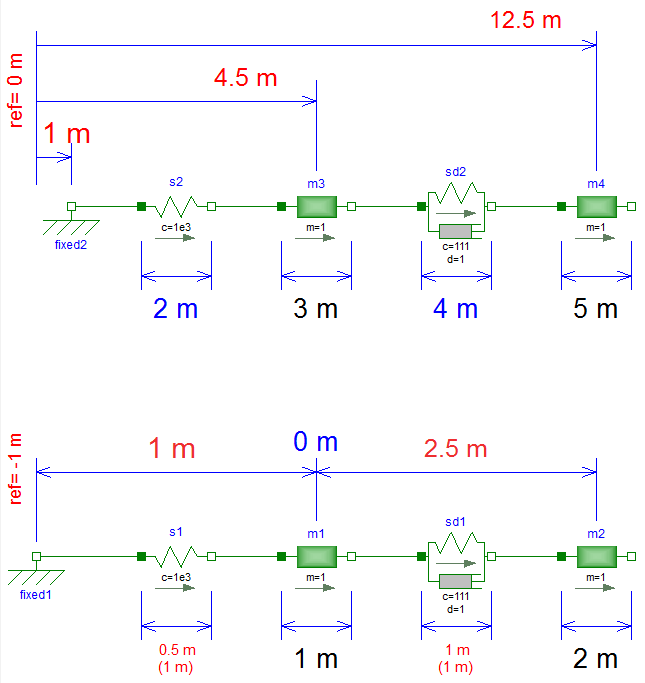# Components (10)

fixed2 s2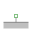Type: Fixed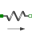Type: Spring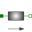Type: Mass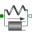Type: SpringDamperType: MassType: FixedType: SpringType: MassType: SpringDamperType: Mass